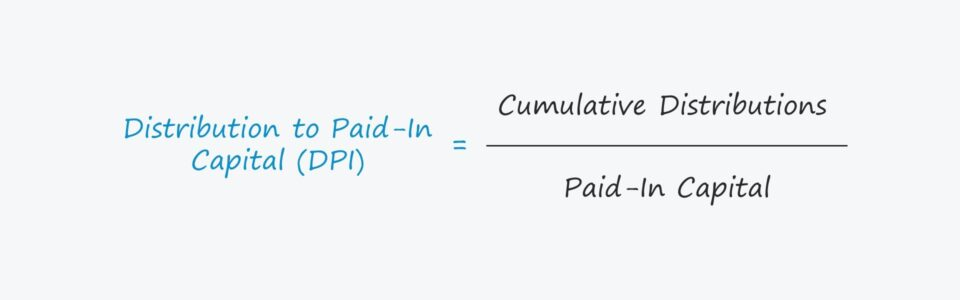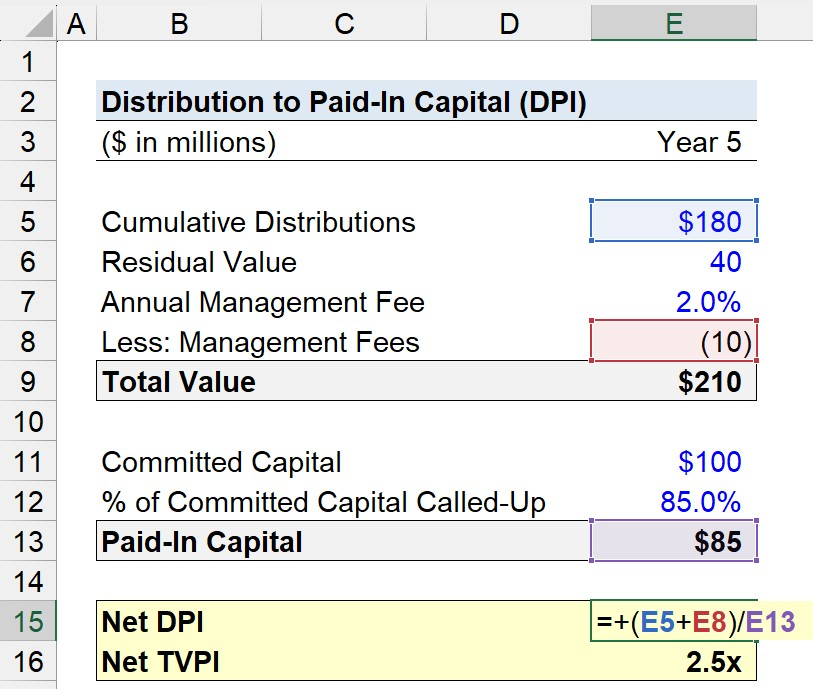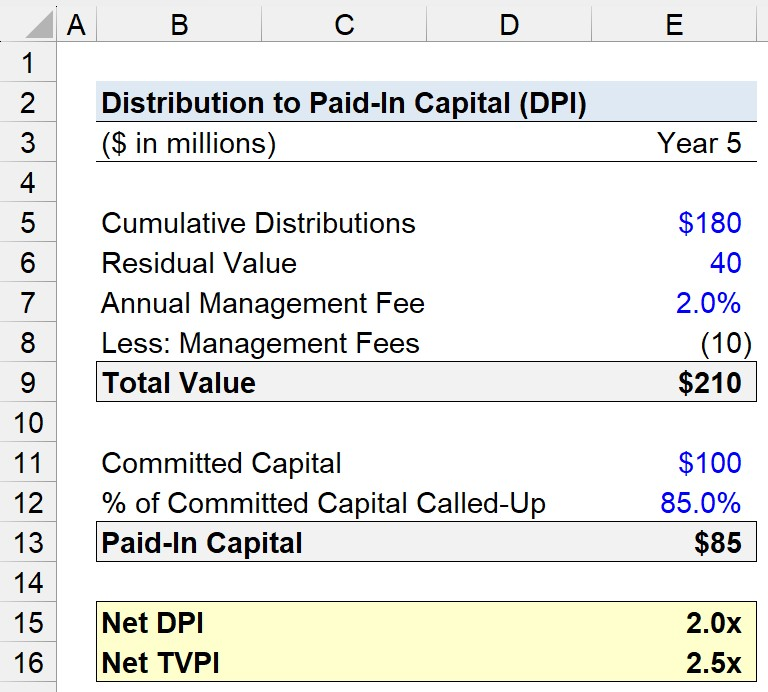Welcome to Wall Street Prep! Use code at checkout for 15% off.# Distribution to Paid-In Capital (DPI)

Guide to Understanding Distribution to Paid-In Capital (DPI)## How to Calculate DPI?

The distribution to paid-in capital metric (DPI) measures the realized profits that have been distributed by the fund back to their limited partners (LPs), i.e. the investor base.

From the perspective of the investor, the DPI metric answers:

• “Given the fund’s called paid-in capital, how much in profits have been realized so far?”

Conceptually, DPI represents the amount actually realized and paid back to investors, so the metric portrays the real profits to date earned by the fund’s limited partners (LPs).

The DPI multiple represents the ratio between the 1) fund’s realized distributions and 2) the paid-in capital of the limited partners (LPs).

• Cumulative Distributions → The total capital returned to LPs (i.e. the realized profits)
• Paid-In Capital → The committed capital from LPs that have been “called” by the investment fund

## DPI Multiple Formula

Calculating the DPI is straightforward, as it involves dividing the realized profits by the capital paid-in by investors.

Distribution to Paid-In Capital (DPI) = Cumulative Distributions ÷ Paid-In Capital

The paid-in capital represents the capital contributed by LPs to the fund that has been “called” by the firm in order to invest it.

The important distinction here is that GPs must make a capital call to the LPs to request access to the committed capital, meaning that paid-in capital is usually NOT equal to the total committed capital amount.

## DPI vs. TVPI Multiple: What is the Difference?

Unlike the total value to paid-in capital (TVPI), the DPI is not inclusive of any residual fund value, i.e. the “paper gains” from investments not yet realized.

At the end of the day, the DPI takes precedence over the TVPI as the fund’s life cycle reaches its later stages and the percentage of committed but uncalled capital remaining is close to zero.

The returns realized once the fund exits investments are true returns, rather than unrealized returns the funds may anticipate on a future exit date.

Hypothetically, if a fund has yet to exit a single investment – neither a full nor partial exit – the DPI amounts to zero.

## What is a Good DPI Multiple?

• DPI Multiple = 1.0x → If a fund’s DPI ratio equals 1.0x precisely, the returned distributions to the investors are equivalent to their paid-in capital.
• DPI Multiple > 1.0x → But if a fund’s DPI ratio exceeds 1.0x, the fund has returned to the LPs all their original paid-in capital (and more) – so, achieving a higher DPI is more beneficial to the firms and their LPs.
• DPI Multiple < 1.0x → Conversely, if a fund’s DPI ratio is below 1.0x, then the fund has failed to return the paid-in capital amount to its investors thus far.

## DPI Multiple Calculator

We’ll now move to a modeling exercise, which you can access by filling out the form below.Submitting...

## 1. Paid-In Capital and Cumulative Distributions Calculation

Suppose a private equity firm has raised a fund with \$100 million in committed capital from their limited partners (LPs).

Of the \$100 million, 85% of the committed capital has been called as of Year 5.

Thus, the paid-in capital equals \$85 million.

• % of Committed Capital Called = 85.0%
• Paid-In Capital = 85.0% × \$100 million = \$85 million

The numerator of the DPI multiple is the cumulative distribution, which we’ll assume to be \$180 million.

• Cumulative Distributions = \$180 million

To have a frame of reference, we’ll also calculate the total value of the paid-in capital (TVPI) multiple.

For the residual value, we’ll assume that the estimated fair value of the unrealized investments is \$40 million.

• Residual Value = \$40 million

## 2. DPI Multiple Calculation Example

For both the DPI and TVPI multiples, the “net” variation will be calculated, so we must account for management fees (and carry, if applicable).

Here, we’ll assume the only expense that affects our return multiples is management fees, which are charged annually at 2.0% of the total committed capital.

• Annual Management Fee = 2.0%
• Management Fees = (2.0% × \$100 million) × 5 Years = \$10 million

The net DPI is calculated by deducting the management fees to date from the cumulative distributions and then dividing that amount by the paid-in capital.

• Net DPI = (\$180 million – \$10 million) ÷ \$85 million = 2.0xTherefore, the net DPI comes out to approximately 2.0x.

In contrast, calculating the net TVPI is conceptually similar, but the notable difference is the inclusion of the residual value – which we’ll assume to be \$40 million.

• Net TVPI = (\$180 million + \$40 million – \$10 million) ÷ \$85 million = 2.5xMaster LBO Modeling Our Advanced LBO Modeling course will teach you how to build a comprehensive LBO model and give you the confidence to ace the finance interview.